cancel
Showing results for
Did you mean:

Fabric is Generally Available. Browse Fabric Presentations. Work towards your Fabric certification with the Cloud Skills Challenge.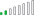Resolver II

## Date of last change

Hello,

I am looking for a solution for my employees' salary analyses report.

I got stuck visualizing the date of the last change (increase) of the following dataset: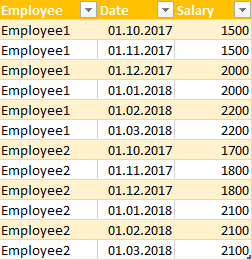What I would like to achieve is the following table:1 ACCEPTED SOLUTIONCommunity Champion

Hi @Birdjo

One way could be a new calculated table

From the Modelling Tab >>>New Table

(Assuming your Table Name is Table1)

```New Table =
VAR temp =
Table1,
"PreviousSalary",
VAR Previous_Date =
CALCULATE (
MAX ( Table1[Date] ),
FILTER (
ALLEXCEPT ( Table1, Table1[Employee] ),
Table1[Date] < EARLIER ( Table1[Date] )
)
)
RETURN
CALCULATE (
SUM ( Table1[Salary] ),
FILTER ( ALLEXCEPT ( Table1, Table1[Employee] ), Table1[Date] = Previous_Date )
)
),
"% increase", Table1[Salary] / [PreviousSalary]
- 1
)
VAR temp2 =
FILTER ( temp, NOT [% increase] IN { 0, 1 / 0 } )
VAR temp3 =
temp2,
"Maxdate", CALCULATE (
MAX ( Table1[Date] ),
FILTER ( temp2, Table1[Employee] = EARLIER ( Table1[Employee] ) )
)
)
VAR Final_Table =
FILTER ( temp3, [Date] = [Maxdate] )
RETURN
Final_Table```

Regards
Zubair

10 REPLIES 10Resolver I

Hi,

You requirement can be achieved through dynamic Measures... which you can modify in a later stage..

I am also addition another column for you ease .. to seee "Previous Salary"

I believe the change % should be wrt to last salary (not wrt latest salary )

Solution pics are mentioend belwo.... Hope you enjoyed it...

Please tick as ans if the solution BAMMED ur prob 😄

I have incorporated all VAR in on measure for your ease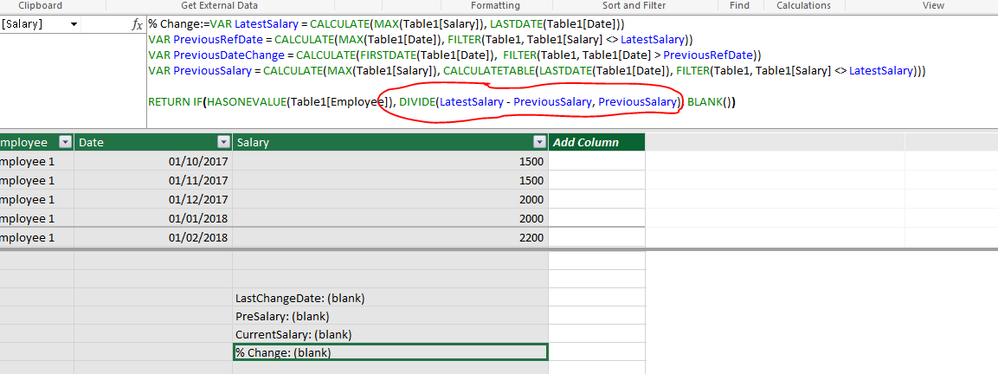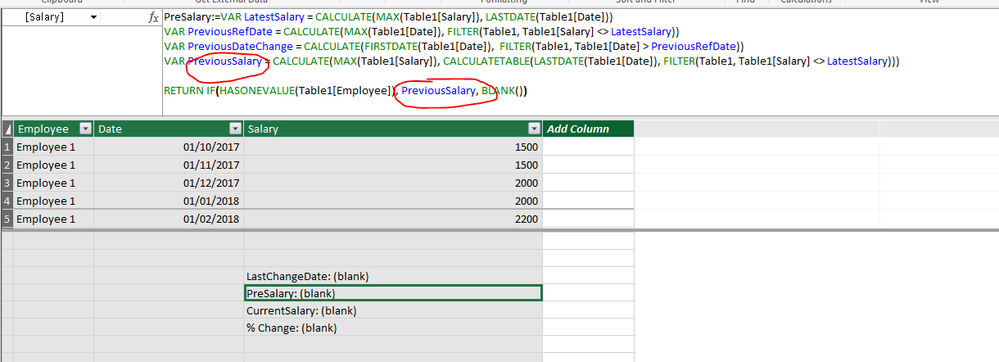Community Champion

@Birdjo

Try this revision

See the attached file as well

```New Table =
VAR temp =
Table1,
"PreviousSalary",
VAR Previous_Date =
CALCULATE (
MAX ( Table1[Date] ),
FILTER (
ALLEXCEPT ( Table1, Table1[Employee] ),
Table1[Date] < EARLIER ( Table1[Date] )
&& Table1[Event] = "Salary"
)
)
RETURN
IF (
Table1[Event] = "Salary",
CALCULATE (
SUM ( Table1[Salary] ),
FILTER (
ALLEXCEPT ( Table1, Table1[Employee] ),
Table1[Date] = Previous_Date
&& Table1[Event] = "Salary"
)
)
)
),
"% increase", Table1[Salary] / [PreviousSalary]
- 1
)
VAR temp2 =
FILTER ( temp, NOT [% increase] IN { 0, 1 / 0 } )
VAR temp3 =
temp2,
"Maxdate", CALCULATE (
MAX ( Table1[Date] ),
FILTER (
temp2,
Table1[Employee] = EARLIER ( Table1[Employee] )
&& Table1[Event] = "Salary"
)
)
)
RETURN
FILTER ( temp3, [Date] = [Maxdate] )```

Regards
ZubairResolver II

I'm sorry I didn't made it clear. The [Event] column is something I write manually just to demonstrate how changes could be something different than a raise. I don't have [Event] column in the original dataset.Community Champion

Hi @Birdjo

This gets really tough thenWhat if we add a calculated column to get the "Event" column

I mean a salary would exist at least 2 or 3 times for an employee...while bonus and raise might be unique figures for one employee

So a calculated column like

```Event =
VAR myCount =
CALCULATE (
COUNTROWS ( Table1 ),
ALLEXCEPT ( Table1, Table1[Employee], Table1[Salary] )
)
RETURN
IF ( myCount > 1, "Salary", "Others" )```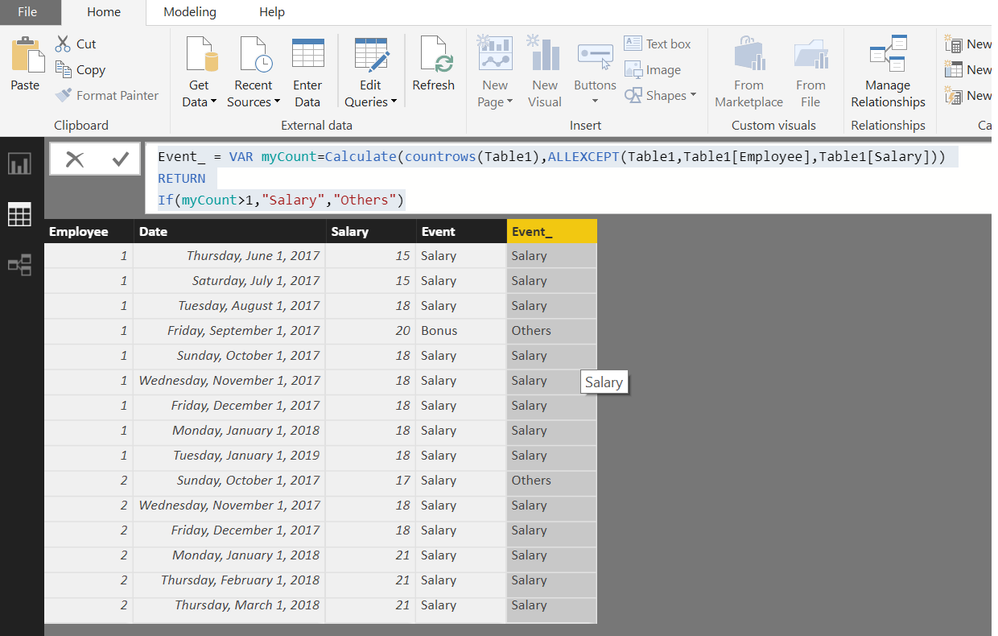Regards
ZubairResolver II

It is really tough indeed. 😄

That's clever but what if the same bonus occurs two times or the raise was in the last month?

It is easy for a human to decide whether it is a raise or something else, however recreating this thought process with DAX seems hard to me.Resolver II

I have some progress with this case.

I have the employee salaries as in their contract. So bonuses are not included there. Only raises and very slight increases and decreases like -2% to +2%.

And I decided to use @Zubair_Muhammad's solution with the calculated table as the other one doesn't behave well because it looks for MAX(Table1[Salary])

Do you have any ideas how to implement some treshold in it?Community Champion

@Birdjo

Hi,Let me try with this:

Assuming you have Every Month Salary of the Employees.

```Variation =
DIVIDE (
Salary[Salary]
- CALCULATE (
MAX ( Salary[Salary] );
FILTER ( Salary; Salary[Employee] = EARLIER ( Salary[Employee] ) );
DATEADD ( Salary[Date]; -1; MONTH )
);
CALCULATE (
MAX ( Salary[Salary] );
FILTER ( Salary; Salary[Employee] = EARLIER ( Salary[Employee] ) );
DATEADD ( Salary[Date]; -1; MONTH )
)
)```

Now, create the measures:

`LastChange = CALCULATE(Max(Salary[Date]);FILTER(Salary;Salary[Variation]<>0 ))`
```MSalary =
VAR LCHANGE = [LastChange]
RETURN
CALCULATE ( MAX ( Salary[Salary] ); FILTER ( Salary; Salary[Date] = LCHANGE ) )```
```M%&Var =
VAR LCHANGE = [LastChange]
RETURN
CALCULATE (
MAX ( Salary[Variation] );
FILTER ( Salary; Salary[Date] = LCHANGE )
)```Regards

Victor

Lima - PeruResolver II

Thanks everybody!

I tested the calculated table and it works fine. However seems like my data isn't that clean and there are some changes in the salaries which are not raises. There 1 month increases such as bonuses and some 1 month decreases in some cases.

I like the calculated column solution although I didn't try it.

Here is more realistic look of my data. The last column called "Event" is something that I wrote manually.In this case the formulas return the last change date as 01.10.2017, however a person can identify that the last raise was on 01.08.2017.

It seems like this case is a serious challenge.
Do you have any ideas how to resolve it?Community Champion

Hi @Birdjo

One way could be a new calculated table

From the Modelling Tab >>>New Table

(Assuming your Table Name is Table1)

```New Table =
VAR temp =
Table1,
"PreviousSalary",
VAR Previous_Date =
CALCULATE (
MAX ( Table1[Date] ),
FILTER (
ALLEXCEPT ( Table1, Table1[Employee] ),
Table1[Date] < EARLIER ( Table1[Date] )
)
)
RETURN
CALCULATE (
SUM ( Table1[Salary] ),
FILTER ( ALLEXCEPT ( Table1, Table1[Employee] ), Table1[Date] = Previous_Date )
)
),
"% increase", Table1[Salary] / [PreviousSalary]
- 1
)
VAR temp2 =
FILTER ( temp, NOT [% increase] IN { 0, 1 / 0 } )
VAR temp3 =
temp2,
"Maxdate", CALCULATE (
MAX ( Table1[Date] ),
FILTER ( temp2, Table1[Employee] = EARLIER ( Table1[Employee] ) )
)
)
VAR Final_Table =
FILTER ( temp3, [Date] = [Maxdate] )
RETURN
Final_Table```

Regards
ZubairCommunity Champion

@BirdjoRegards
ZubairAnnouncements#### Power BI Monthly Update - November 2023

Check out the November 2023 Power BI update to learn about new features.#### Fabric Community News unified experience

Read the latest Fabric Community announcements, including updates on Power BI, Synapse, Data Factory and Data Activator.#### The largest Power BI and Fabric virtual conference

130+ sessions, 130+ speakers, Product managers, MVPs, and experts. All about Power BI and Fabric. Attend online or watch the recordings.Top Solution Authors
Top Kudoed Authors
Users online (4,263)